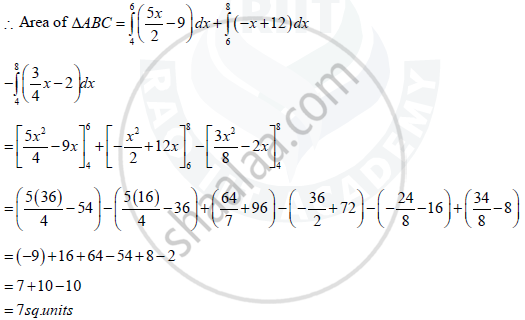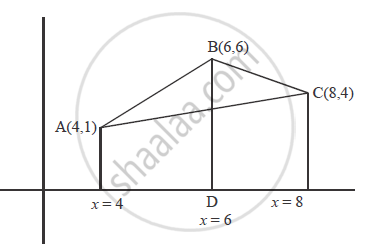# Using the Method of Integration, Find the Area of the Triangle Abc, Coordinates of Whose Vertices Are a (4 , 1), B (6, 6) and C (8, 4). - Mathematics

Using the method of integration, find the area of the triangle ABC, coordinates of whose vertices are A (4 , 1), B (6, 6) and C (8, 4).

#### Solution

Equation of AB

y - y_1 = (y_2 -y_1)/(x_2-x_1) (x - x_1)

y - 1 = (6-1)/(6-4) (x - 4)

y - 1 = 5/2 (x - 4)

2y - 2 = 5x - 20

y = (5x)/2 - 9

Equation of BC

y - 6 = (4 - 6)/(8 - 6) (x - 6)

y - 6 = (-2)/(+2) (x - 6)

y - 6 = -x + 6

y = -x + 12

Equation of AC

y - 1 = (4 -1)/(8 - 4) (x - 4)

y -1 = 3/4 (x - 4)

4y - 4 = 3x - 12

y = (3x)/4 - 2Concept: Area Under Simple Curves
Is there an error in this question or solution?